Скачать презентацию Social Science Statistics Module I Gwilym Pryce Lecture

357595361be23985cd03e37ee6e0f181.ppt

• Количество слайдов: 54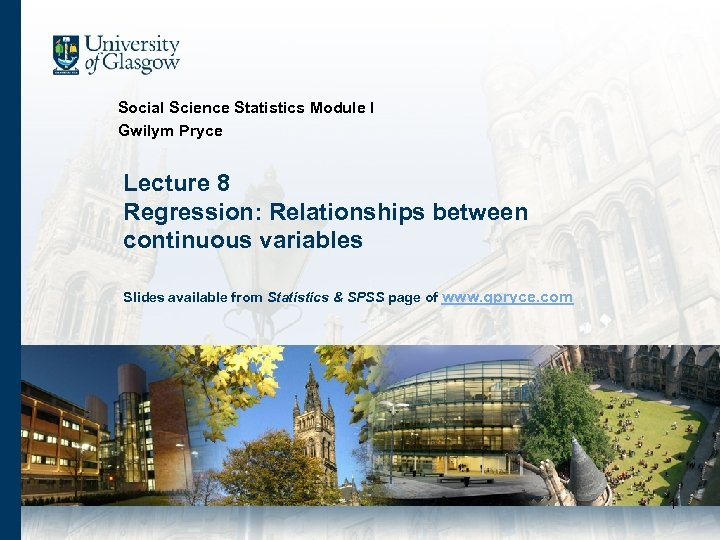Social Science Statistics Module I Gwilym Pryce Lecture 8 Regression: Relationships between continuous variables Slides available from Statistics & SPSS page of www. gpryce. com 1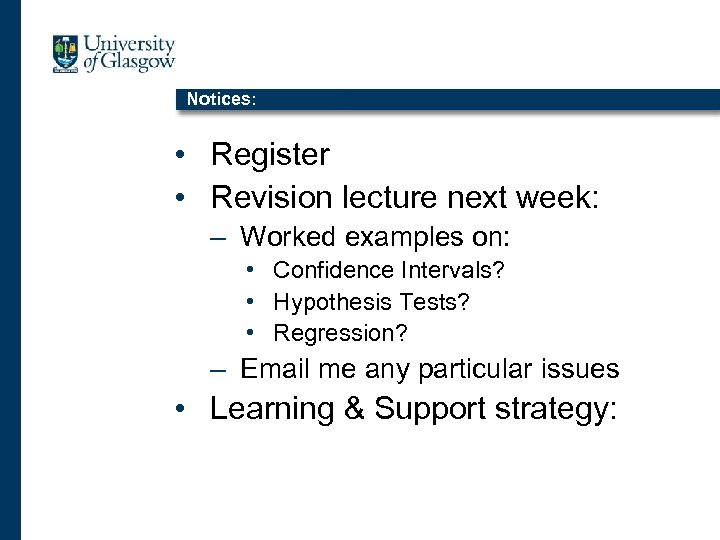Notices: • Register • Revision lecture next week: – Worked examples on: • Confidence Intervals? • Hypothesis Tests? • Regression? – Email me any particular issues • Learning & Support strategy: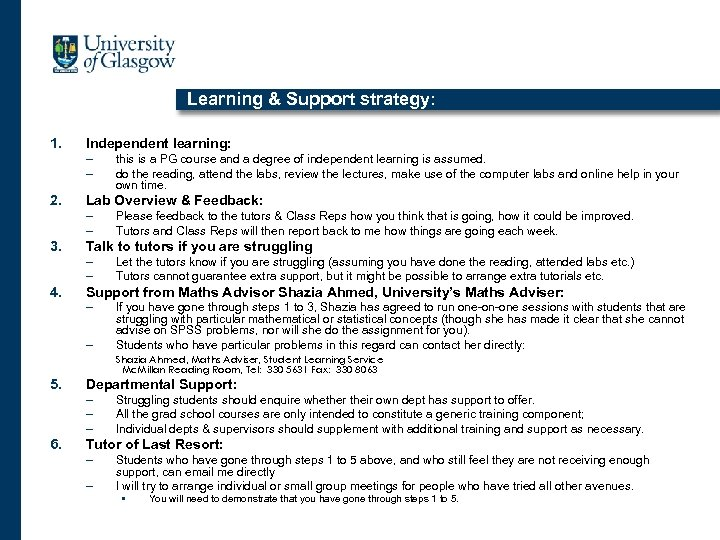Learning & Support strategy: 1. Independent learning: – – 2. Lab Overview & Feedback: – – 3. Please feedback to the tutors & Class Reps how you think that is going, how it could be improved. Tutors and Class Reps will then report back to me how things are going each week. Talk to tutors if you are struggling – – 4. this is a PG course and a degree of independent learning is assumed. do the reading, attend the labs, review the lectures, make use of the computer labs and online help in your own time. Let the tutors know if you are struggling (assuming you have done the reading, attended labs etc. ) Tutors cannot guarantee extra support, but it might be possible to arrange extra tutorials etc. Support from Maths Advisor Shazia Ahmed, University’s Maths Adviser: – – If you have gone through steps 1 to 3, Shazia has agreed to run one-on-one sessions with students that are struggling with particular mathematical or statistical concepts (though she has made it clear that she cannot advise on SPSS problems, nor will she do the assignment for you). Students who have particular problems in this regard can contact her directly: Shazia Ahmed, Maths Adviser, Student Learning Service Mc. Millan Reading Room, Tel: 330 5631 Fax: 330 8063 5. Departmental Support: – – – 6. Struggling students should enquire whether their own dept has support to offer. All the grad school courses are only intended to constitute a generic training component; Individual depts & supervisors should supplement with additional training and support as necessary. Tutor of Last Resort: – – Students who have gone through steps 1 to 5 above, and who still feel they are not receiving enough support, can email me directly I will try to arrange individual or small group meetings for people who have tried all other avenues. • You will need to demonstrate that you have gone through steps 1 to 5.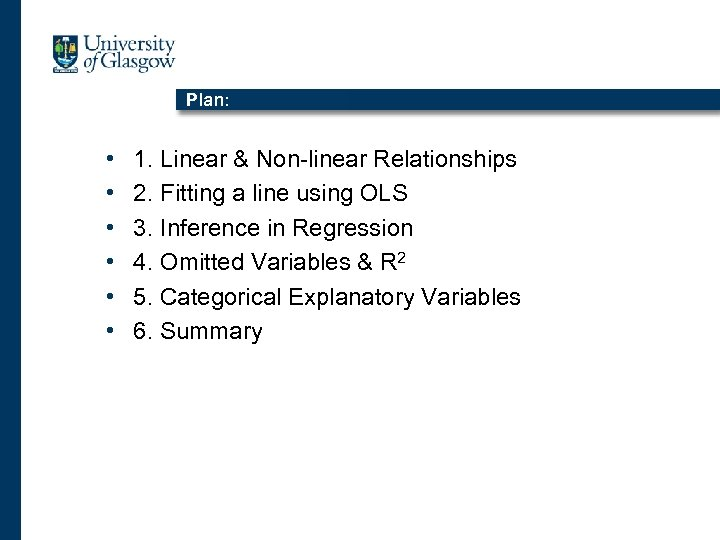Plan: • • • 1. Linear & Non-linear Relationships 2. Fitting a line using OLS 3. Inference in Regression 4. Omitted Variables & R 2 5. Categorical Explanatory Variables 6. Summary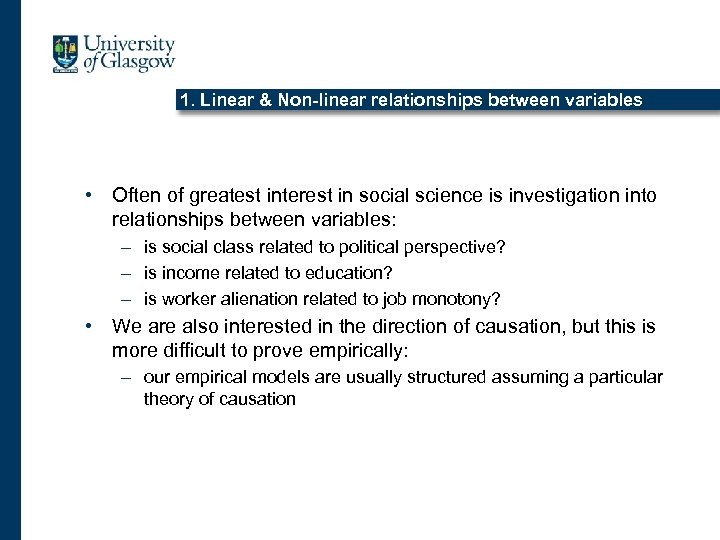1. Linear & Non-linear relationships between variables • Often of greatest interest in social science is investigation into relationships between variables: – is social class related to political perspective? – is income related to education? – is worker alienation related to job monotony? • We are also interested in the direction of causation, but this is more difficult to prove empirically: – our empirical models are usually structured assuming a particular theory of causation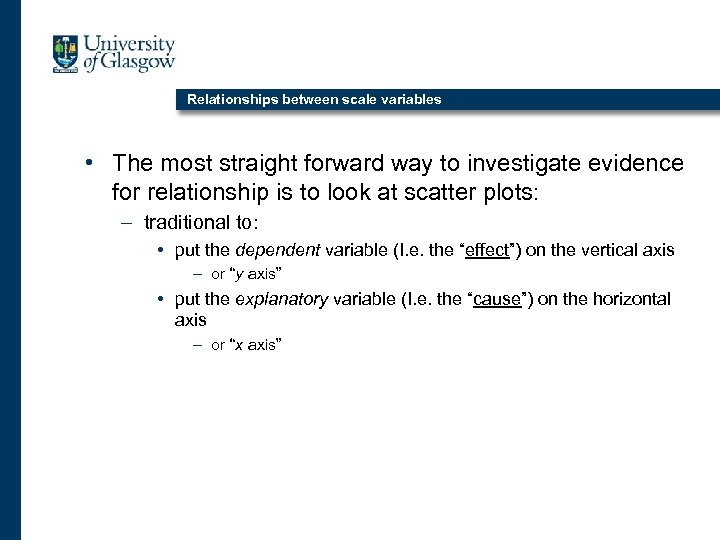Relationships between scale variables • The most straight forward way to investigate evidence for relationship is to look at scatter plots: – traditional to: • put the dependent variable (I. e. the “effect”) on the vertical axis – or “y axis” • put the explanatory variable (I. e. the “cause”) on the horizontal axis – or “x axis”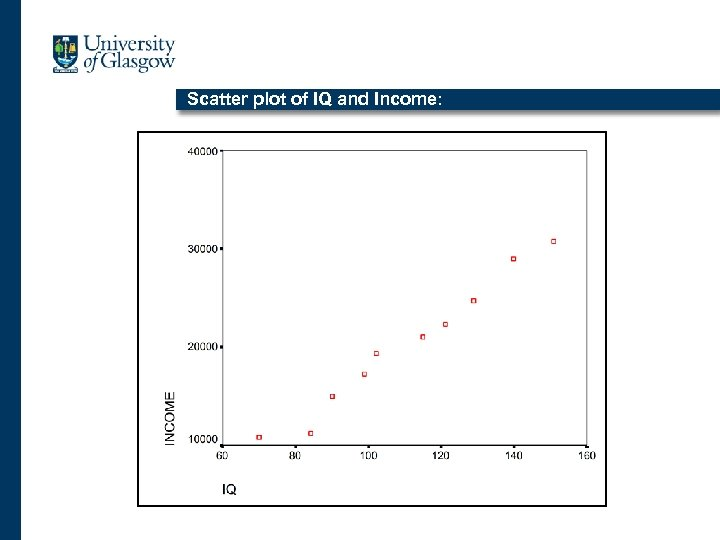Scatter plot of IQ and Income: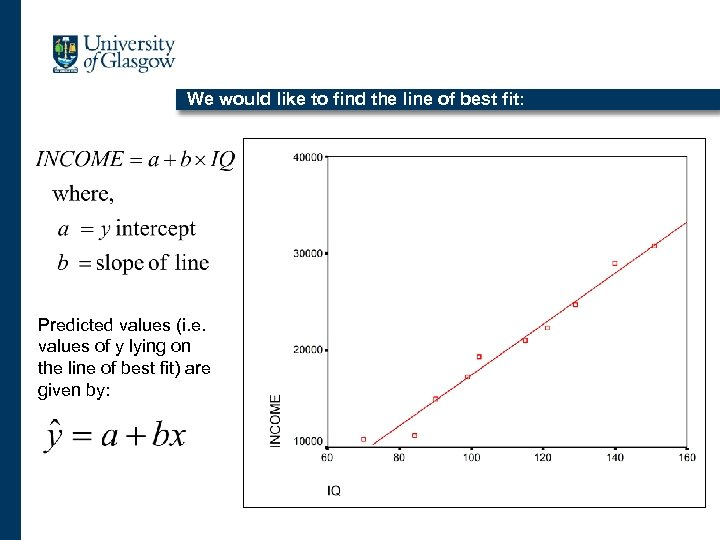We would like to find the line of best fit: Predicted values (i. e. values of y lying on the line of best fit) are given by: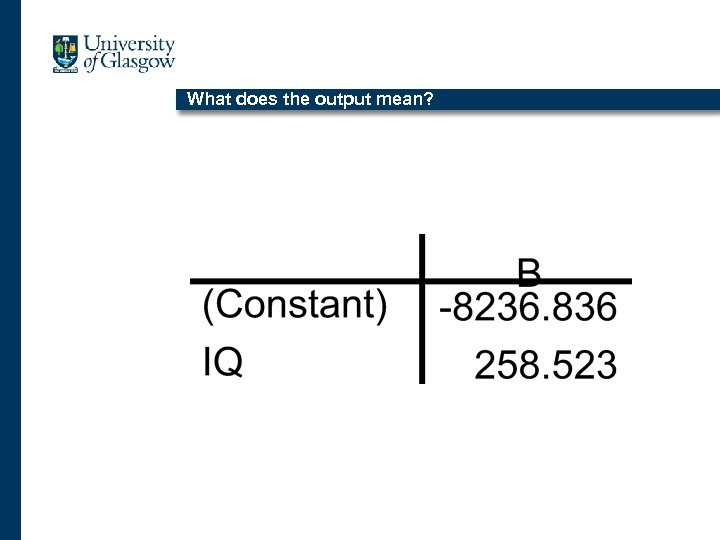What does the output mean?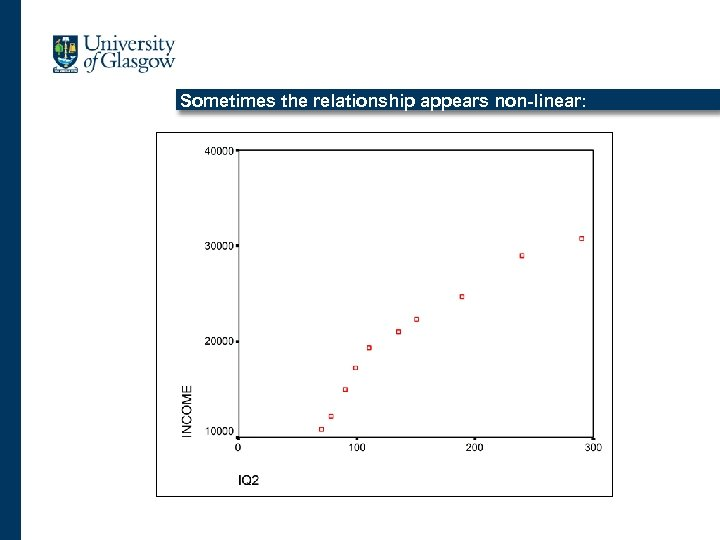Sometimes the relationship appears non-linear: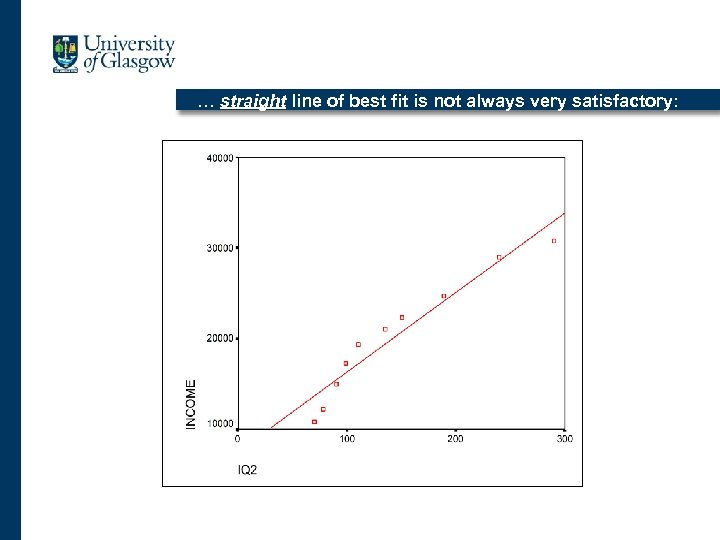… straight line of best fit is not always very satisfactory: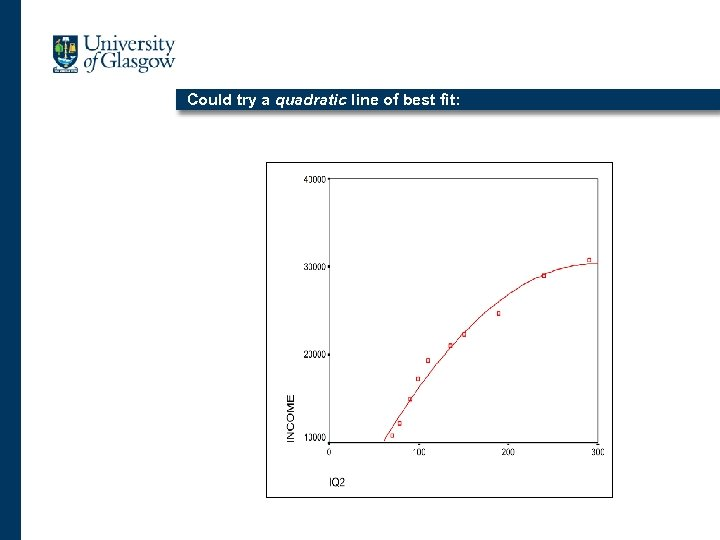Could try a quadratic line of best fit: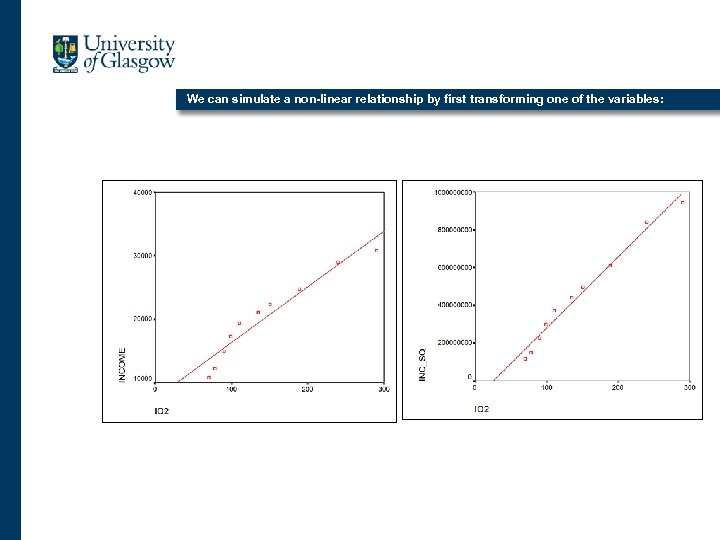We can simulate a non-linear relationship by first transforming one of the variables: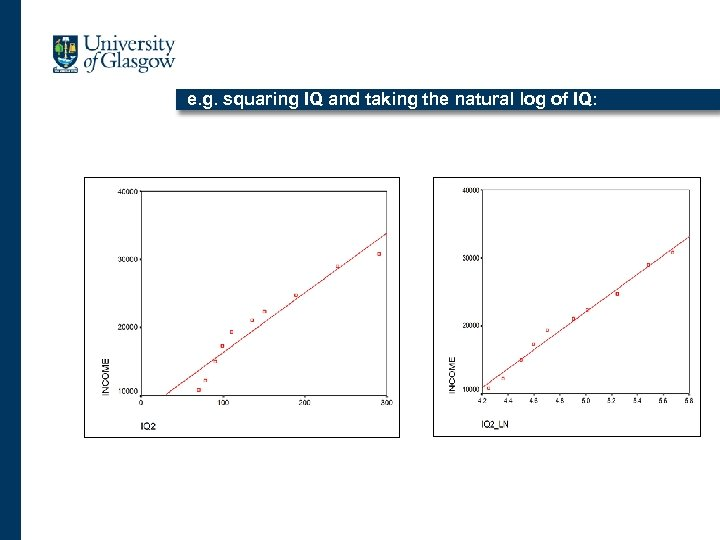e. g. squaring IQ and taking the natural log of IQ: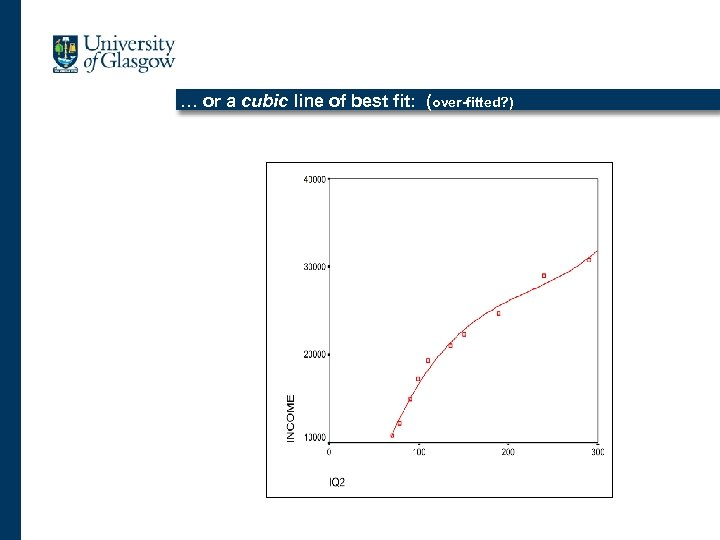… or a cubic line of best fit: (over-fitted? )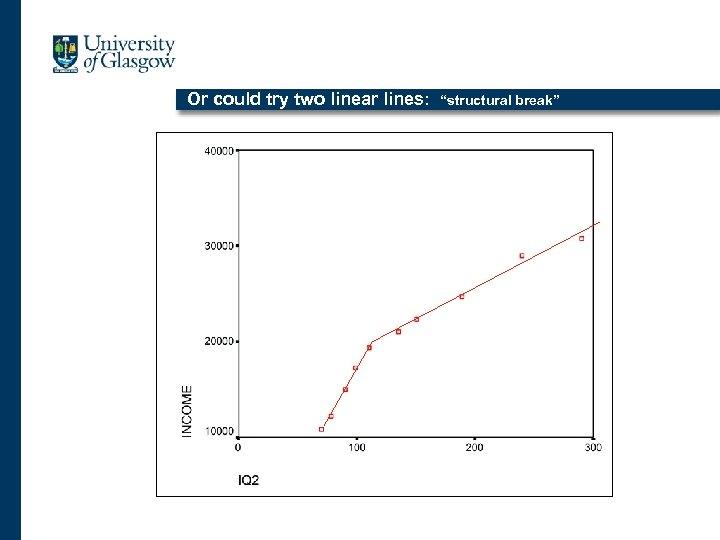Or could try two linear lines: “structural break”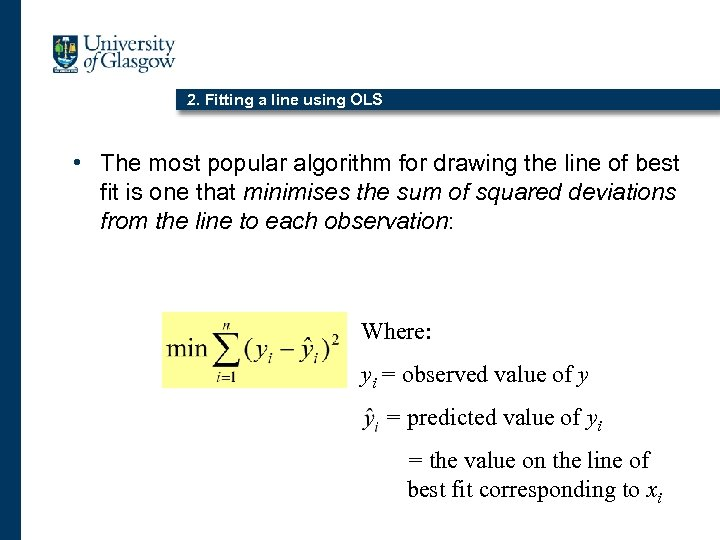2. Fitting a line using OLS • The most popular algorithm for drawing the line of best fit is one that minimises the sum of squared deviations from the line to each observation: Where: yi = observed value of y = predicted value of yi = the value on the line of best fit corresponding to xi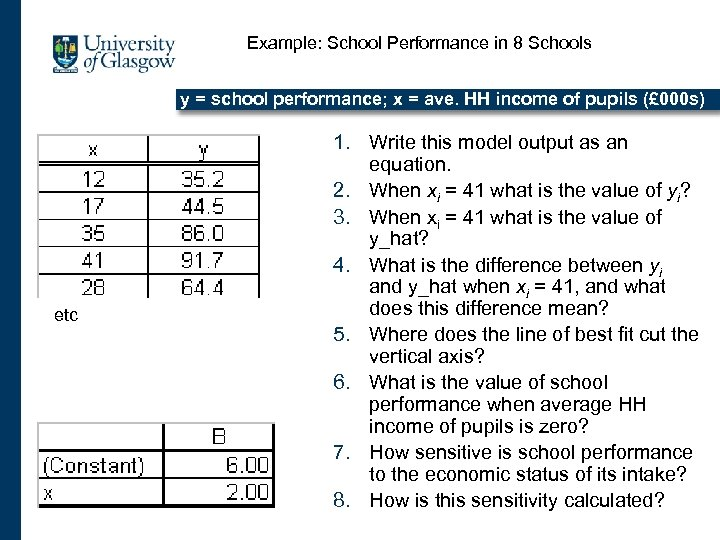Example: School Performance in 8 Schools y = school performance; x = ave. HH income of pupils (£ 000 s) etc 1. Write this model output as an equation. 2. When xi = 41 what is the value of yi? 3. When xi = 41 what is the value of y_hat? 4. What is the difference between yi and y_hat when xi = 41, and what does this difference mean? 5. Where does the line of best fit cut the vertical axis? 6. What is the value of school performance when average HH income of pupils is zero? 7. How sensitive is school performance to the economic status of its intake? 8. How is this sensitivity calculated?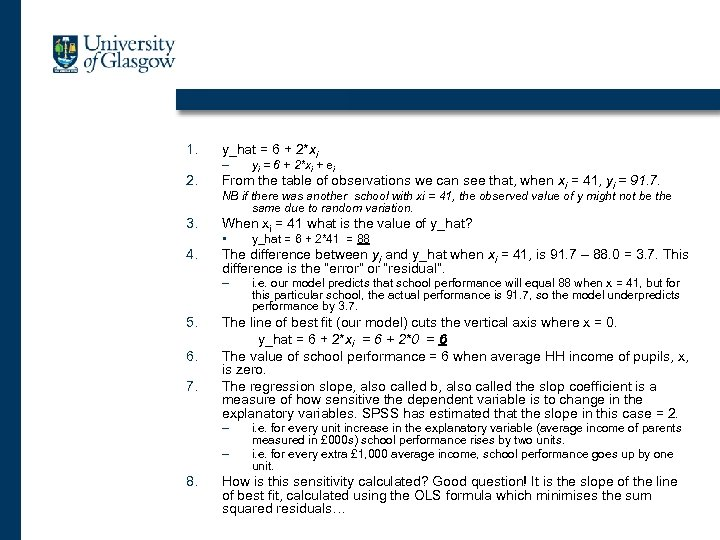1. y_hat = 6 + 2*xi – 2. yi = 6 + 2*xi + ei From the table of observations we can see that, when xi = 41, yi = 91. 7. NB if there was another school with xi = 41, the observed value of y might not be the same due to random variation. 3. When xi = 41 what is the value of y_hat? • 4. The difference between yi and y_hat when xi = 41, is 91. 7 – 88. 0 = 3. 7. This difference is the “error” or “residual”. – 5. 6. 7. i. e. our model predicts that school performance will equal 88 when x = 41, but for this particular school, the actual performance is 91. 7, so the model underpredicts performance by 3. 7. The line of best fit (our model) cuts the vertical axis where x = 0. y_hat = 6 + 2*xi = 6 + 2*0 = 6 The value of school performance = 6 when average HH income of pupils, x, is zero. The regression slope, also called b, also called the slop coefficient is a measure of how sensitive the dependent variable is to change in the explanatory variables. SPSS has estimated that the slope in this case = 2. – – 8. y_hat = 6 + 2*41 = 88 i. e. for every unit increase in the explanatory variable (average income of parents measured in £ 000 s) school performance rises by two units. i. e. for every extra £ 1, 000 average income, school performance goes up by one unit. How is this sensitivity calculated? Good question! It is the slope of the line of best fit, calculated using the OLS formula which minimises the sum squared residuals…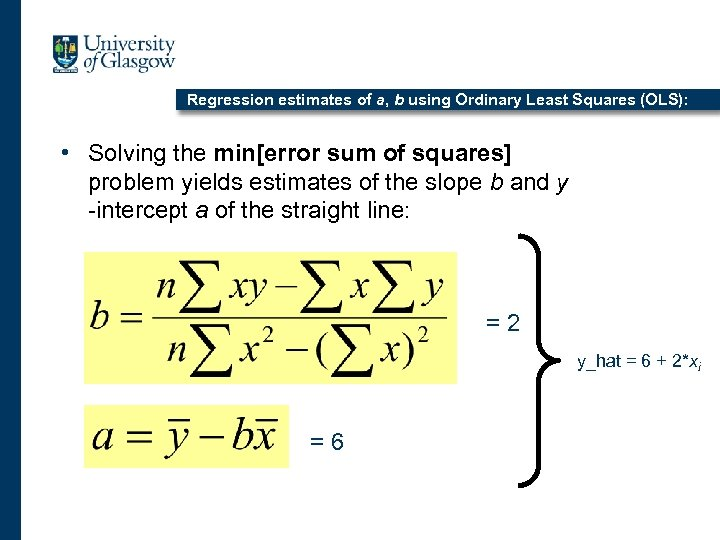Regression estimates of a, b using Ordinary Least Squares (OLS): • Solving the min[error sum of squares] problem yields estimates of the slope b and y -intercept a of the straight line: =2 y_hat = 6 + 2*xi =6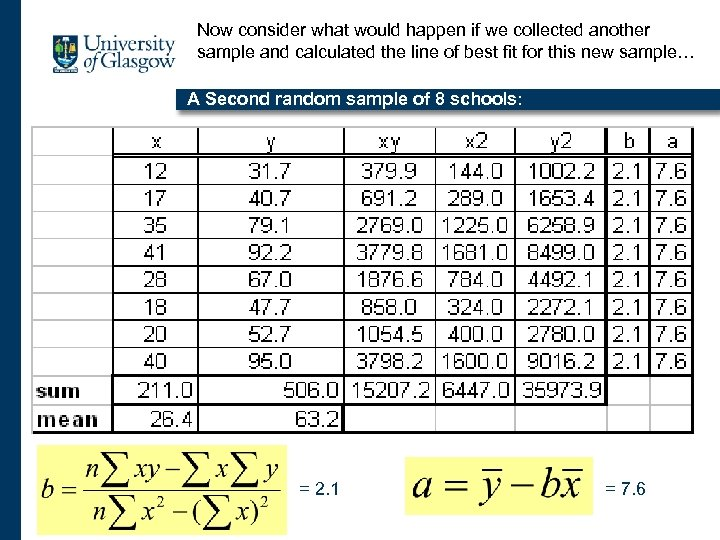Now consider what would happen if we collected another sample and calculated the line of best fit for this new sample… A Second random sample of 8 schools: = 2. 1 = 7. 6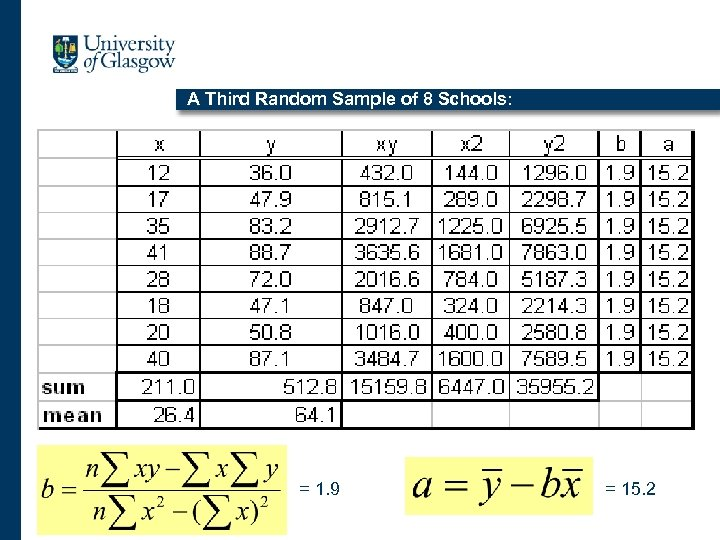A Third Random Sample of 8 Schools: = 1. 9 = 15. 2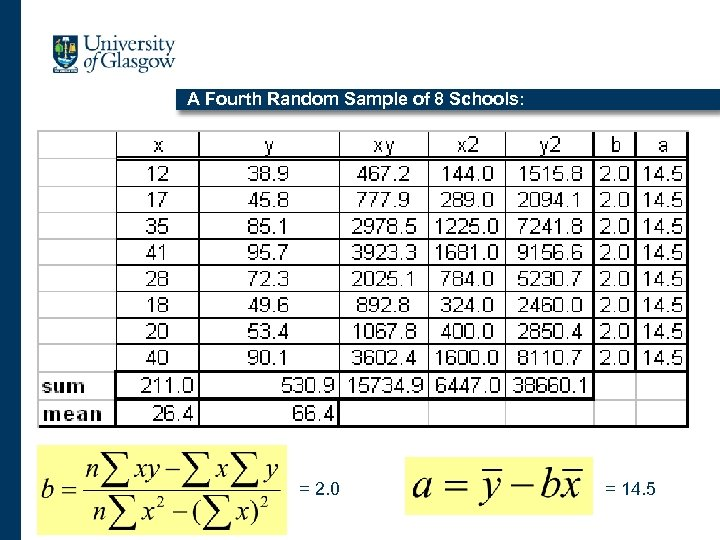A Fourth Random Sample of 8 Schools: = 2. 0 = 14. 5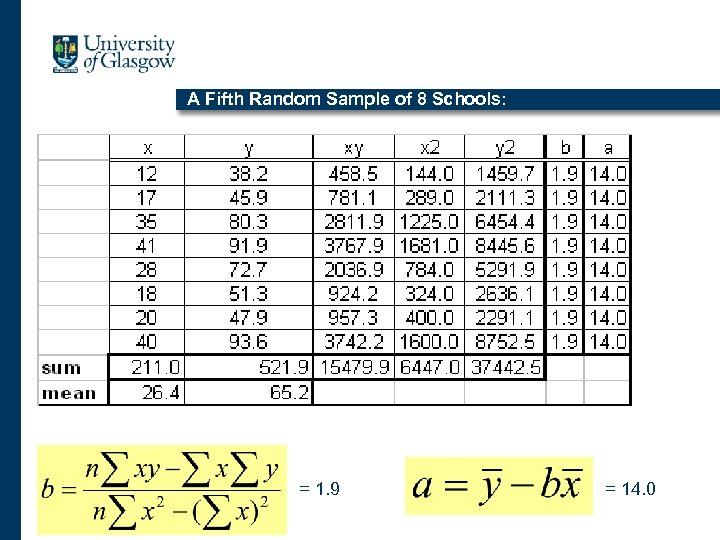A Fifth Random Sample of 8 Schools: = 1. 9 = 14. 0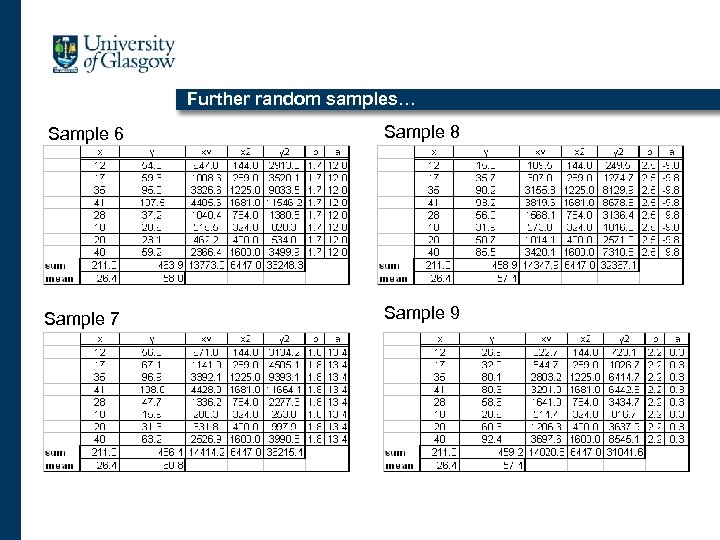Further random samples… Sample 6 Sample 8 Sample 7 Sample 9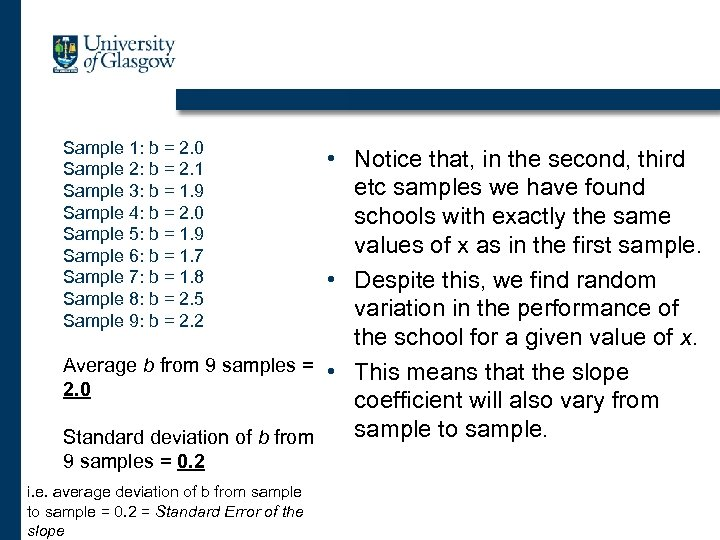Sample 1: b = 2. 0 Sample 2: b = 2. 1 Sample 3: b = 1. 9 Sample 4: b = 2. 0 Sample 5: b = 1. 9 Sample 6: b = 1. 7 Sample 7: b = 1. 8 Sample 8: b = 2. 5 Sample 9: b = 2. 2 • Notice that, in the second, third etc samples we have found schools with exactly the same values of x as in the first sample. • Despite this, we find random variation in the performance of the school for a given value of x. Average b from 9 samples = • This means that the slope 2. 0 coefficient will also vary from sample to sample. Standard deviation of b from 9 samples = 0. 2 i. e. average deviation of b from sample to sample = 0. 2 = Standard Error of the slope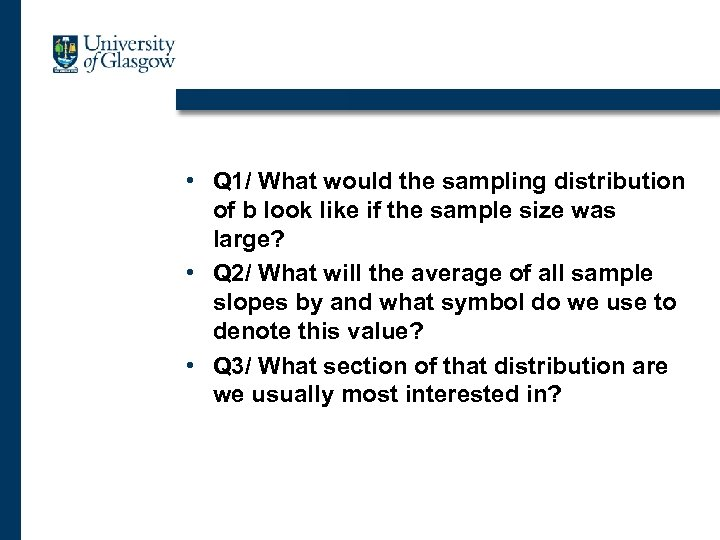• Q 1/ What would the sampling distribution of b look like if the sample size was large? • Q 2/ What will the average of all sample slopes by and what symbol do we use to denote this value? • Q 3/ What section of that distribution are we usually most interested in?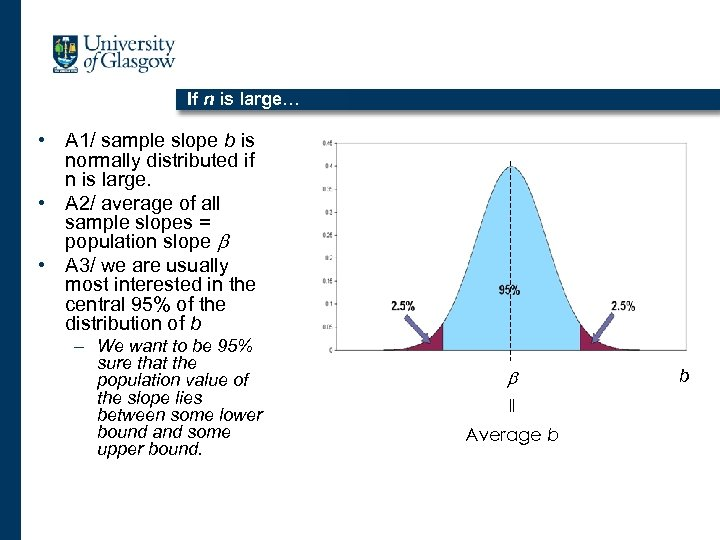If n is large… • A 1/ sample slope b is normally distributed if n is large. • A 2/ average of all sample slopes = population slope b • A 3/ we are usually most interested in the central 95% of the distribution of b – We want to be 95% sure that the population value of the slope lies between some lower bound and some upper bound. b || Average b b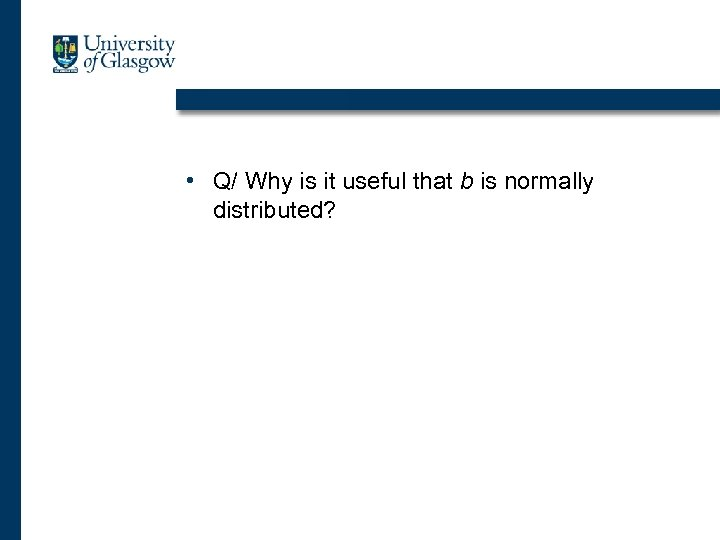• Q/ Why is it useful that b is normally distributed?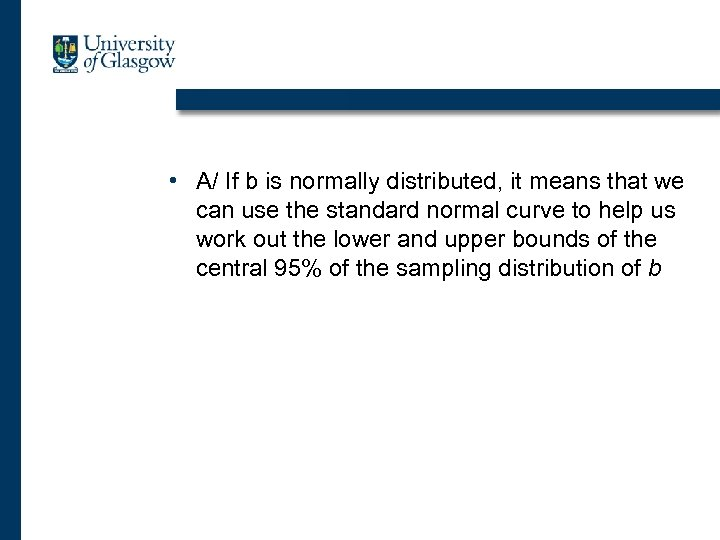• A/ If b is normally distributed, it means that we can use the standard normal curve to help us work out the lower and upper bounds of the central 95% of the sampling distribution of b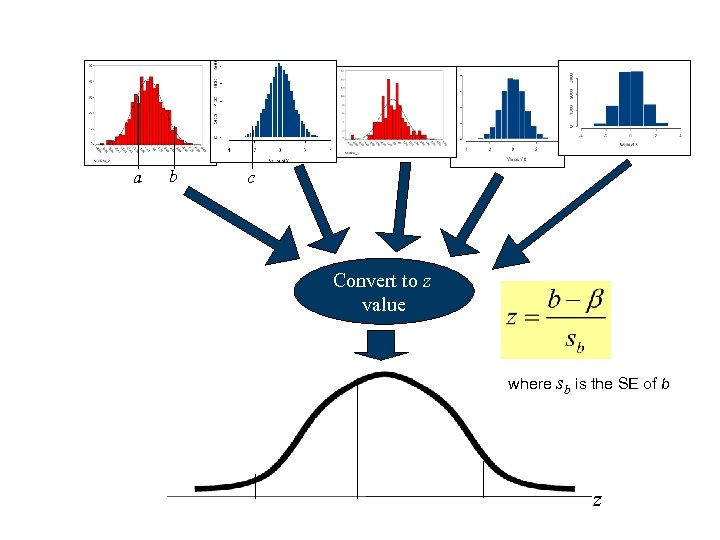a b c Convert to z value where sb is the SE of b z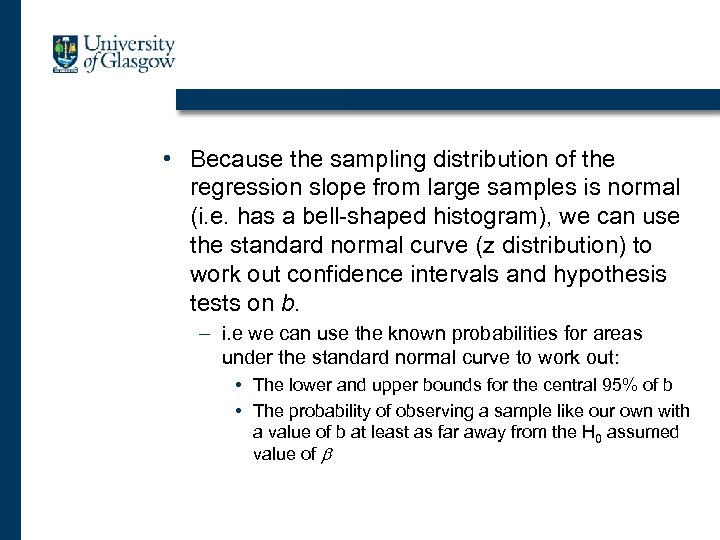• Because the sampling distribution of the regression slope from large samples is normal (i. e. has a bell-shaped histogram), we can use the standard normal curve (z distribution) to work out confidence intervals and hypothesis tests on b. – i. e we can use the known probabilities for areas under the standard normal curve to work out: • The lower and upper bounds for the central 95% of b • The probability of observing a sample like our own with a value of b at least as far away from the H 0 assumed value of b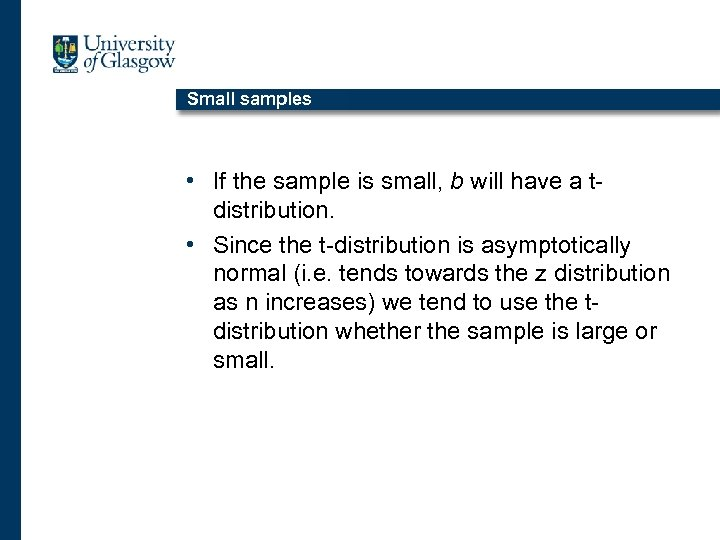Small samples • If the sample is small, b will have a tdistribution. • Since the t-distribution is asymptotically normal (i. e. tends towards the z distribution as n increases) we tend to use the tdistribution whether the sample is large or small.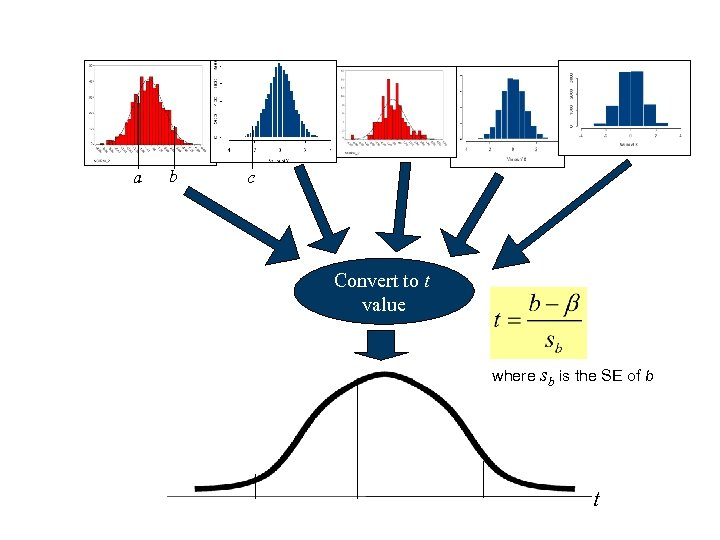a b c Convert to t value where sb is the SE of b t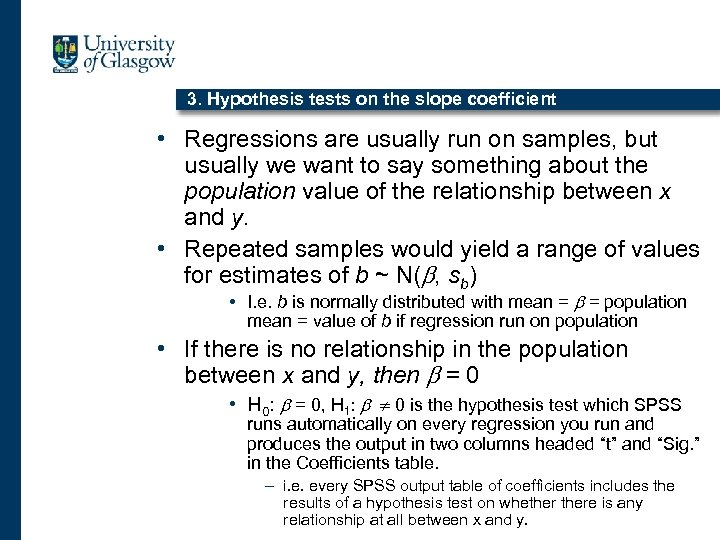3. Hypothesis tests on the slope coefficient • Regressions are usually run on samples, but usually we want to say something about the population value of the relationship between x and y. • Repeated samples would yield a range of values for estimates of b ~ N(b, sb) • I. e. b is normally distributed with mean = b = population mean = value of b if regression run on population • If there is no relationship in the population between x and y, then b = 0 • H 0: b = 0, H 1: b 0 is the hypothesis test which SPSS runs automatically on every regression you run and produces the output in two columns headed “t” and “Sig. ” in the Coefficients table. – i. e. every SPSS output table of coefficients includes the results of a hypothesis test on whethere is any relationship at all between x and y.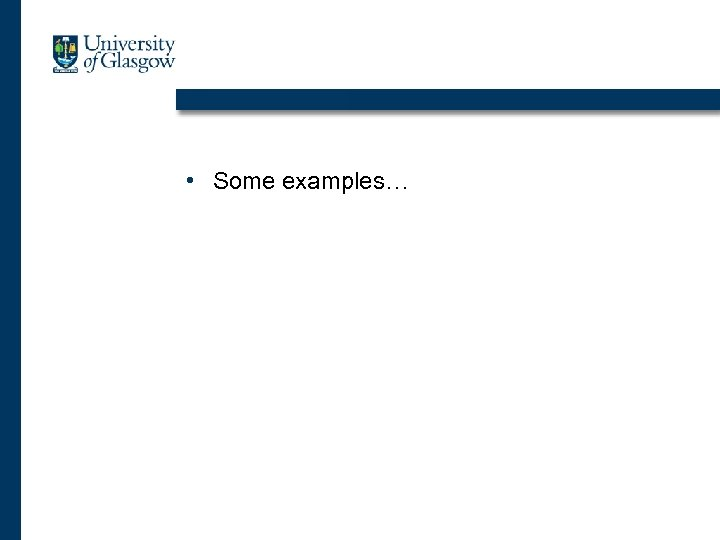• Some examples…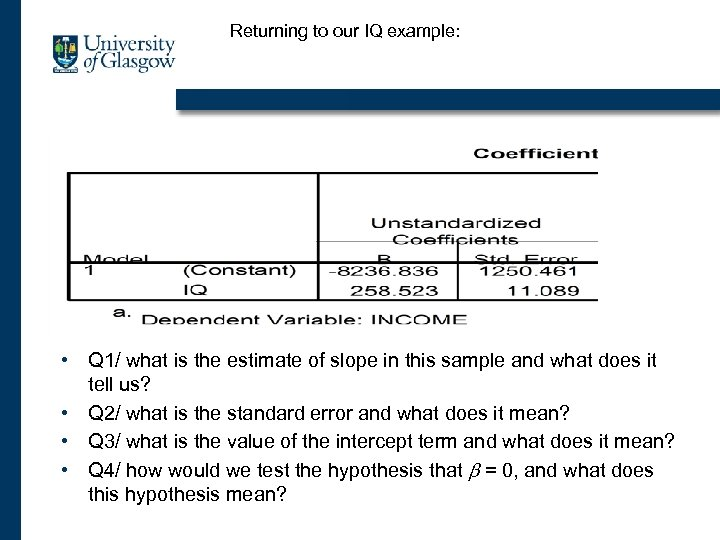Returning to our IQ example: • Q 1/ what is the estimate of slope in this sample and what does it tell us? • Q 2/ what is the standard error and what does it mean? • Q 3/ what is the value of the intercept term and what does it mean? • Q 4/ how would we test the hypothesis that b = 0, and what does this hypothesis mean?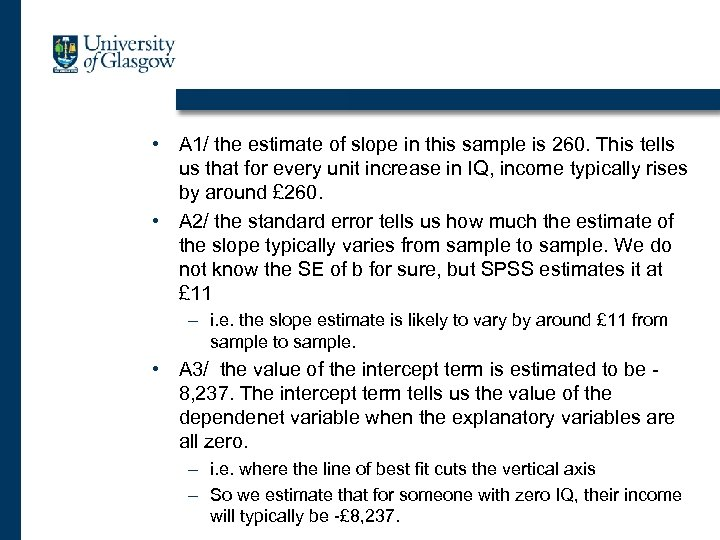• A 1/ the estimate of slope in this sample is 260. This tells us that for every unit increase in IQ, income typically rises by around £ 260. • A 2/ the standard error tells us how much the estimate of the slope typically varies from sample to sample. We do not know the SE of b for sure, but SPSS estimates it at £ 11 – i. e. the slope estimate is likely to vary by around £ 11 from sample to sample. • A 3/ the value of the intercept term is estimated to be 8, 237. The intercept term tells us the value of the dependenet variable when the explanatory variables are all zero. – i. e. where the line of best fit cuts the vertical axis – So we estimate that for someone with zero IQ, their income will typically be -£ 8, 237.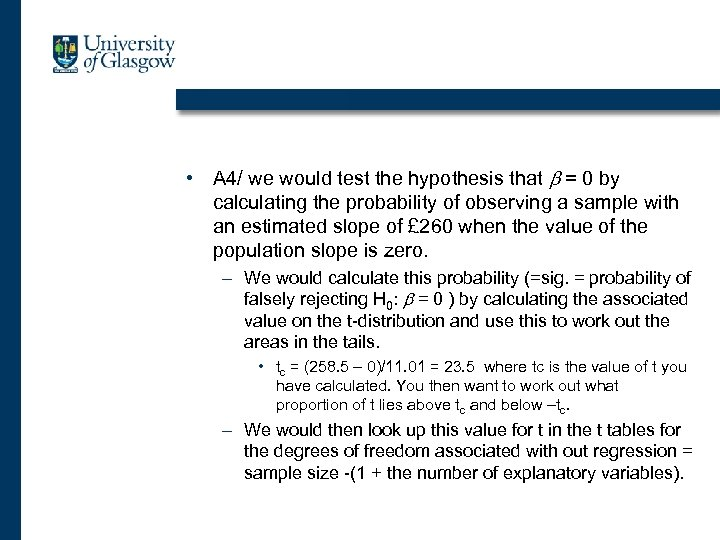• A 4/ we would test the hypothesis that b = 0 by calculating the probability of observing a sample with an estimated slope of £ 260 when the value of the population slope is zero. – We would calculate this probability (=sig. = probability of falsely rejecting H 0: b = 0 ) by calculating the associated value on the t-distribution and use this to work out the areas in the tails. • tc = (258. 5 – 0)/11. 01 = 23. 5 where tc is the value of t you have calculated. You then want to work out what proportion of t lies above tc and below –tc. – We would then look up this value for t in the t tables for the degrees of freedom associated with out regression = sample size -(1 + the number of explanatory variables).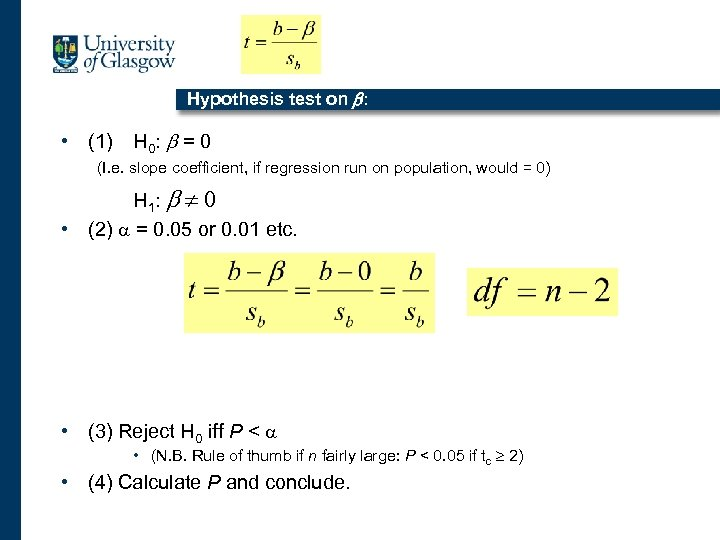Hypothesis test on b: • (1) H 0 : b = 0 (I. e. slope coefficient, if regression run on population, would = 0) H 1: b 0 • (2) a = 0. 05 or 0. 01 etc. • (3) Reject H 0 iff P < a • (N. B. Rule of thumb if n fairly large: P < 0. 05 if tc 2) • (4) Calculate P and conclude.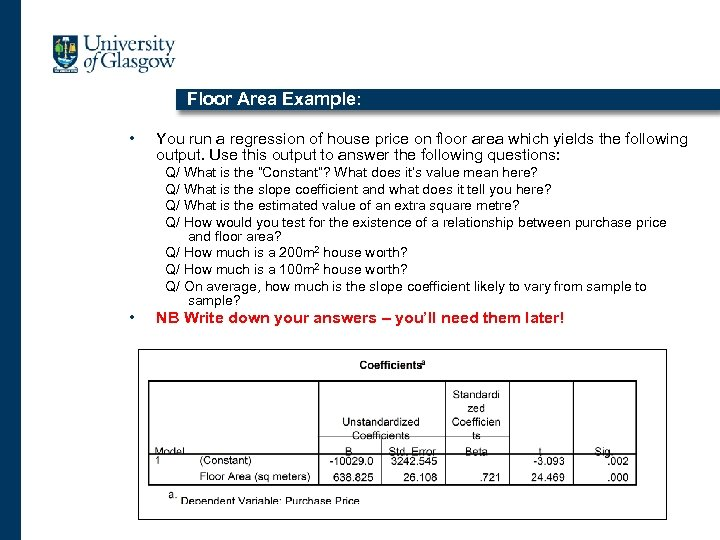Floor Area Example: • You run a regression of house price on floor area which yields the following output. Use this output to answer the following questions: Q/ What is the “Constant”? What does it’s value mean here? Q/ What is the slope coefficient and what does it tell you here? Q/ What is the estimated value of an extra square metre? Q/ How would you test for the existence of a relationship between purchase price and floor area? Q/ How much is a 200 m 2 house worth? Q/ How much is a 100 m 2 house worth? Q/ On average, how much is the slope coefficient likely to vary from sample to sample? • NB Write down your answers – you’ll need them later!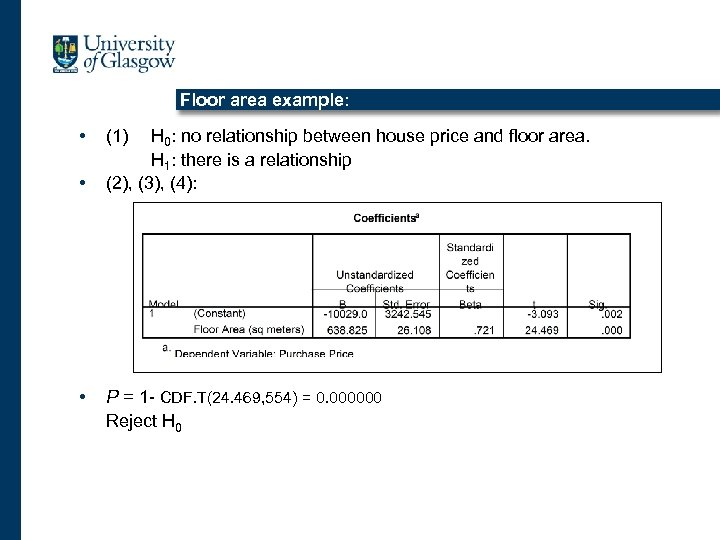Floor area example: • • • (1) H 0: no relationship between house price and floor area. H 1: there is a relationship (2), (3), (4): P = 1 - CDF. T(24. 469, 554) = 0. 000000 Reject H 0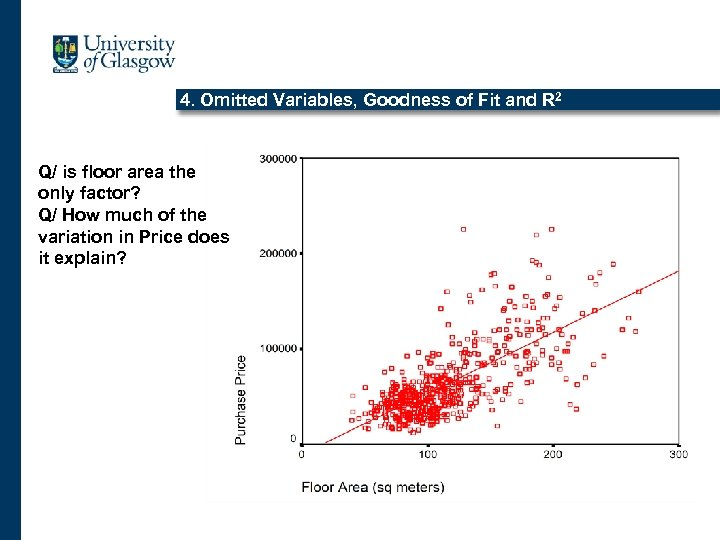4. Omitted Variables, Goodness of Fit and R 2 Q/ is floor area the only factor? Q/ How much of the variation in Price does it explain?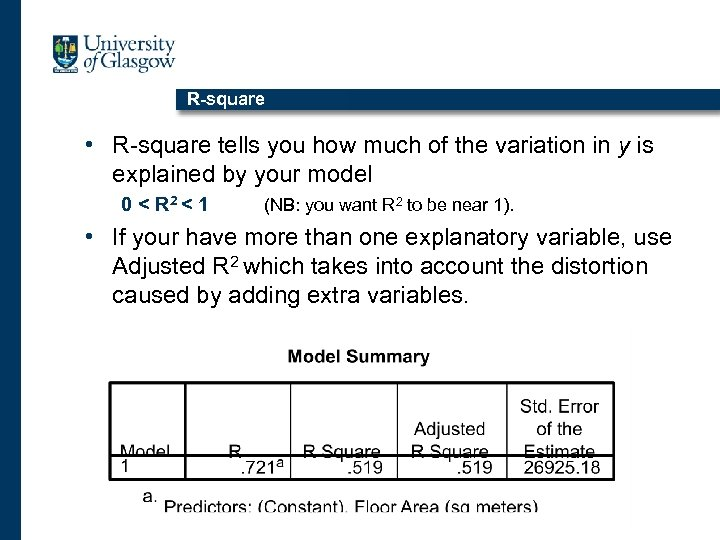R-square • R-square tells you how much of the variation in y is explained by your model 0 < R 2 < 1 (NB: you want R 2 to be near 1). • If your have more than one explanatory variable, use Adjusted R 2 which takes into account the distortion caused by adding extra variables.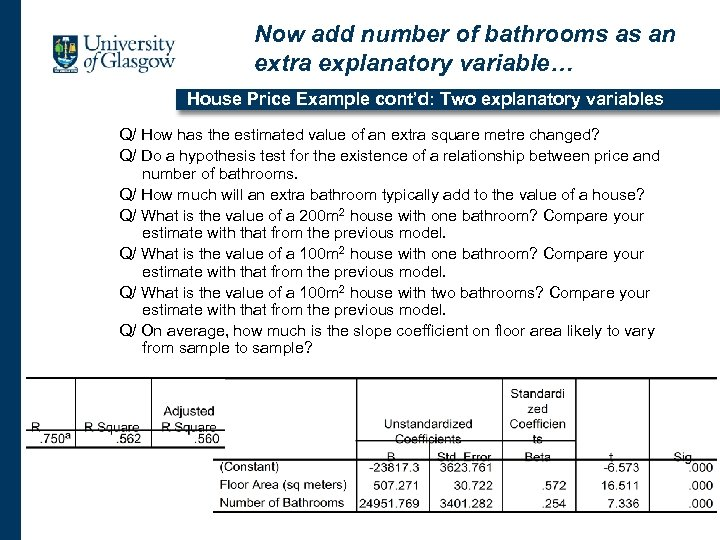Now add number of bathrooms as an extra explanatory variable… House Price Example cont’d: Two explanatory variables Q/ How has the estimated value of an extra square metre changed? Q/ Do a hypothesis test for the existence of a relationship between price and number of bathrooms. Q/ How much will an extra bathroom typically add to the value of a house? Q/ What is the value of a 200 m 2 house with one bathroom? Compare your estimate with that from the previous model. Q/ What is the value of a 100 m 2 house with two bathrooms? Compare your estimate with that from the previous model. Q/ On average, how much is the slope coefficient on floor area likely to vary from sample to sample?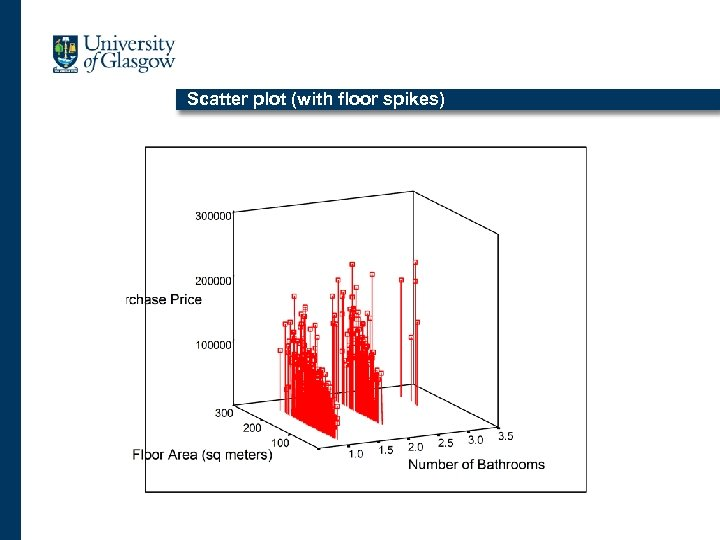Scatter plot (with floor spikes)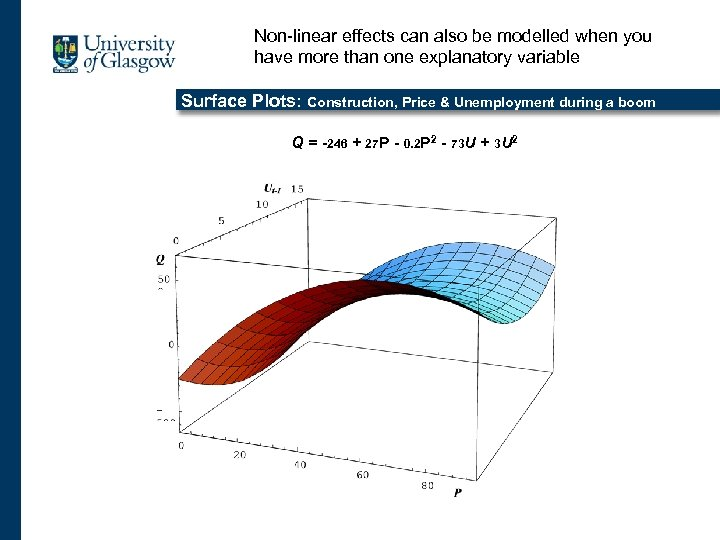Non-linear effects can also be modelled when you have more than one explanatory variable 3 D Surface Plots: Construction, Price & Unemployment during a boom Q = -246 + 27 P - 0. 2 P 2 - 73 U + 3 U 2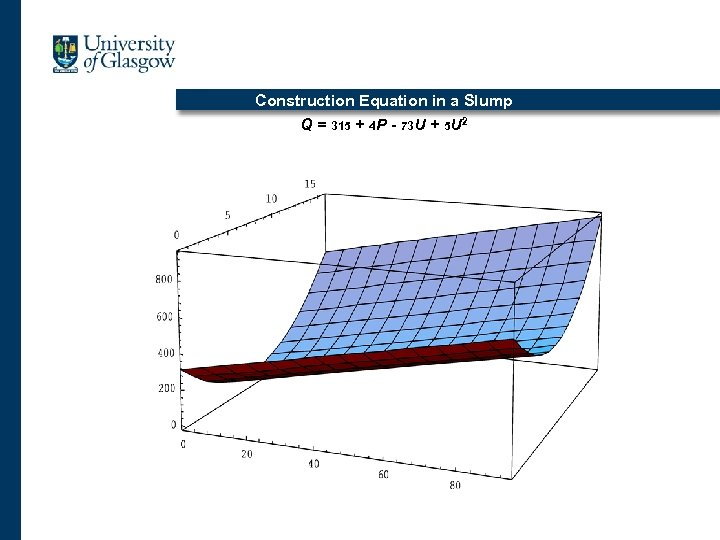Construction Equation in a Slump Q = 315 + 4 P - 73 U + 5 U 2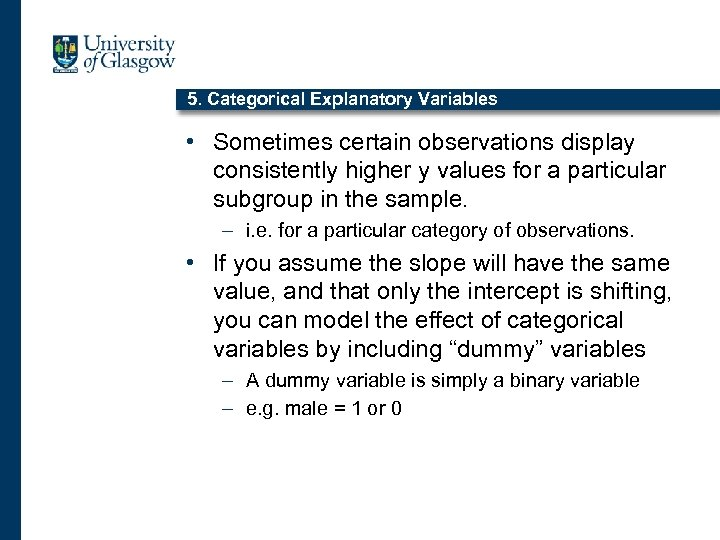5. Categorical Explanatory Variables • Sometimes certain observations display consistently higher y values for a particular subgroup in the sample. – i. e. for a particular category of observations. • If you assume the slope will have the same value, and that only the intercept is shifting, you can model the effect of categorical variables by including “dummy” variables – A dummy variable is simply a binary variable – e. g. male = 1 or 0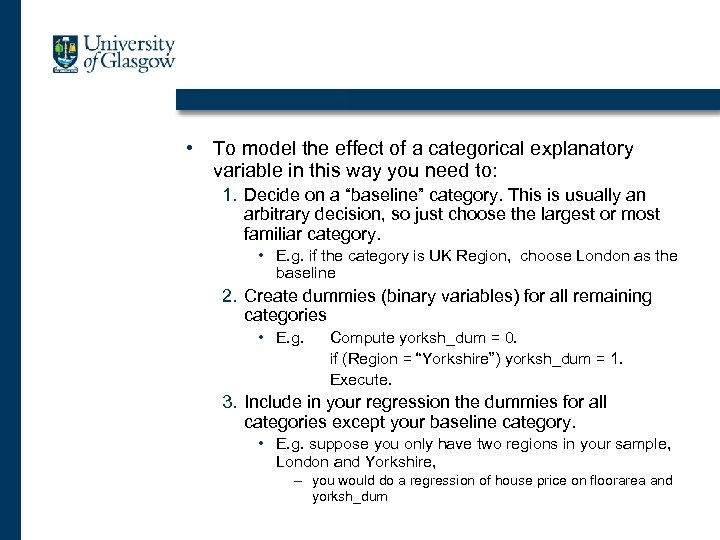• To model the effect of a categorical explanatory variable in this way you need to: 1. Decide on a “baseline” category. This is usually an arbitrary decision, so just choose the largest or most familiar category. • E. g. if the category is UK Region, choose London as the baseline 2. Create dummies (binary variables) for all remaining categories • E. g. Compute yorksh_dum = 0. if (Region = “Yorkshire”) yorksh_dum = 1. Execute. 3. Include in your regression the dummies for all categories except your baseline category. • E. g. suppose you only have two regions in your sample, London and Yorkshire, – you would do a regression of house price on floorarea and yorksh_dum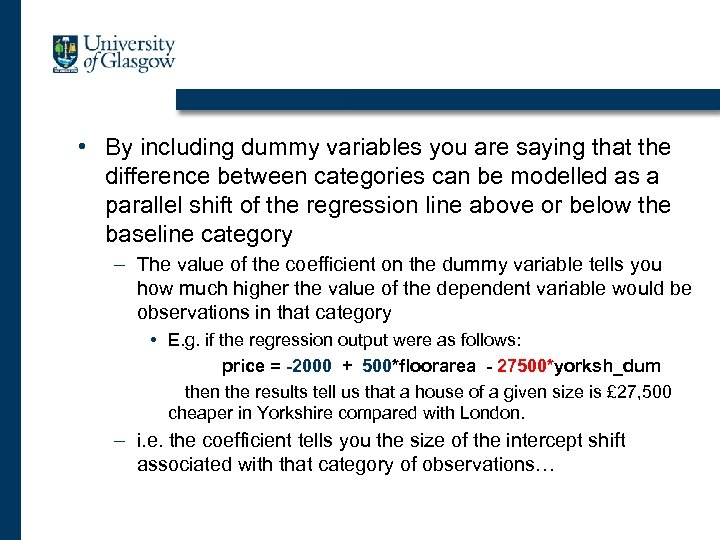• By including dummy variables you are saying that the difference between categories can be modelled as a parallel shift of the regression line above or below the baseline category – The value of the coefficient on the dummy variable tells you how much higher the value of the dependent variable would be observations in that category • E. g. if the regression output were as follows: price = -2000 + 500*floorarea - 27500*yorksh_dum then the results tell us that a house of a given size is £ 27, 500 cheaper in Yorkshire compared with London. – i. e. the coefficient tells you the size of the intercept shift associated with that category of observations…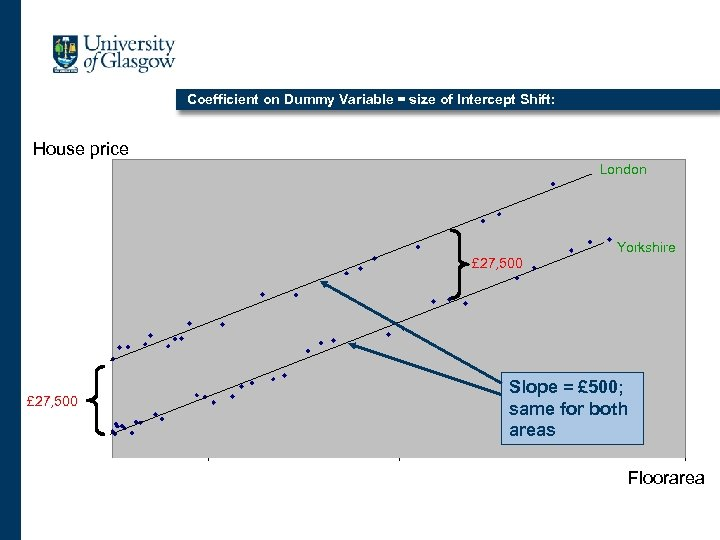Coefficient on Dummy Variable = size of Intercept Shift: House price London Yorkshire £ 27, 500 Slope = £ 500; same for both areas Floorarea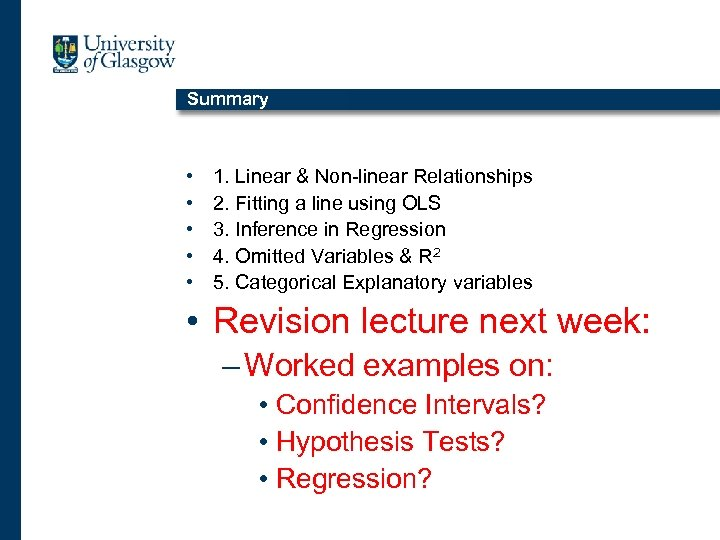Summary • • • 1. Linear & Non-linear Relationships 2. Fitting a line using OLS 3. Inference in Regression 4. Omitted Variables & R 2 5. Categorical Explanatory variables • Revision lecture next week: – Worked examples on: • Confidence Intervals? • Hypothesis Tests? • Regression?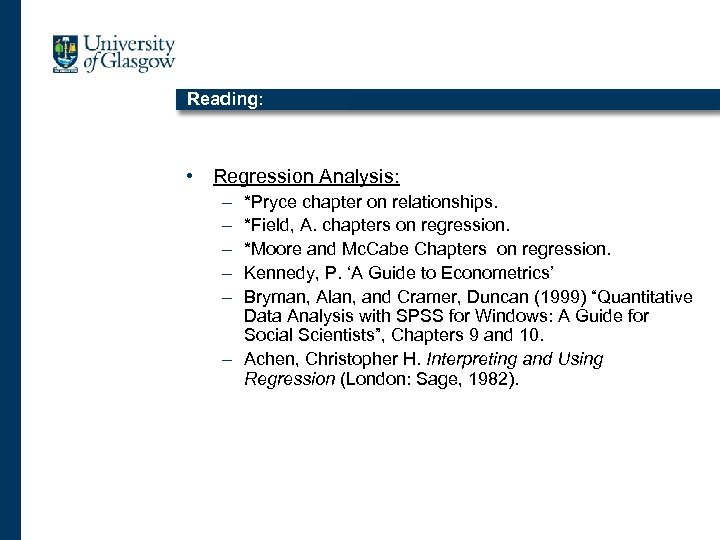Reading: • Regression Analysis: – – – *Pryce chapter on relationships. *Field, A. chapters on regression. *Moore and Mc. Cabe Chapters on regression. Kennedy, P. ‘A Guide to Econometrics’ Bryman, Alan, and Cramer, Duncan (1999) “Quantitative Data Analysis with SPSS for Windows: A Guide for Social Scientists”, Chapters 9 and 10. – Achen, Christopher H. Interpreting and Using Regression (London: Sage, 1982).